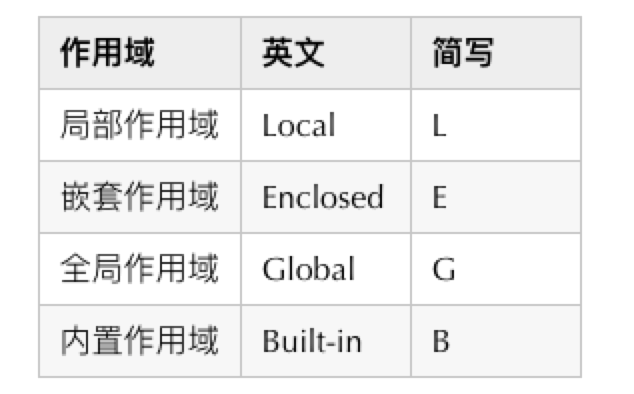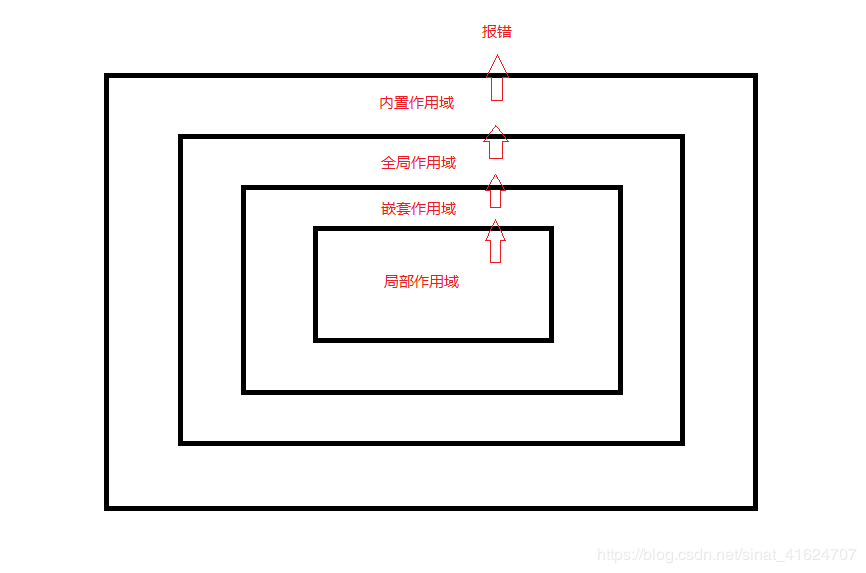Python 的四种作用域及调用顺序Young先生﻿

局部作用域#

Copydef myfun():
a = 0 # 局部变量
a += 1
print("myfun a = ", a)
def myfun2():
a = [1, 2, 3] # 局部变量
a = [i + 1 for i in a]
print("myfun2 a = ", a)
a = 'Hello Python' # 此处a为全局变量
myfun()
myfun2()
print("函数外 a = ", a)

myfun a = 1
myfun2 a = [2, 3, 4]

嵌套作用域#

Copy# 1.函数嵌套：
def myfun(i):
a = [1, 2, 3] # a 所在范围为嵌套作用域
a.append(i)
return a
test = myfun(4)
print(test())

﻿

Copy# 2.类中包含多个函数
class test:
b = 1
def __init__(self):
self.a = 0
def myfun2(self):
self.a += 1
print("a = ", self.a)
self.b += 1
print("b = ", self.b)
test = test()
test.myfun2()

a = 1
b = 2

全局作用域#

Copy# 整数，字符串等不可更改
a = 1 # a为全局变量
def myfun():
a = 2 # 局部变量
a += 1
print("函数中 a = ", a)
myfun()
print("函数外 a = ", a)

﻿

Copy# 列表，元组等可更改
a = [1, 2, 3]
b = {'语文': 98, "数学": 101}
def myfun():
a.append(4)
b.update({"英语": 103})
print("函数中 a = ", a)
print("函数中 b = ", b)
myfun()
print("函数外 a = ", a)
print("函数外 b = ", b)

﻿

Copy# 利用global关键字 引用全局变量
a = 1
def myfun():
global a # 使用a之前用global
a += 1
print("函数中 a = ", a)
myfun()
print("函数外 a = ", a)

总结#

python的四种所用域及其调用顺序，当使用某一变量时，首先在函数内部进行搜索（局部作用域 L），搜索不到便到上层函数或类中进行查找（嵌套作用域 E），再查找不到便到更上层模块中进行查找（全局作用域 G）,还查找不到则进入预先定义的（内置作用域 B）中查找，查找不到报错。Young先生

Python工程师/书虫/极客/ 2020.04.22 加入

评论